# Grade - math word problems

#### Number of problems found: 5415

• Complementary angles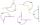Two angles are complementary. If one angle measures 10°, what is the measure of the second angle?
• Rabbits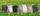In the hutch are 48 mottled rabbits. Brown are 23 less than mottled and white are 8-times less than mottled. How many rabbits are in the hutch?
• Pairs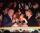At the table sit 10 people, 5 on one side and 5 on the other side. Among them are 3 pairs. Every pair wants to sit opposite each other. How many ways can they sit?
• Scale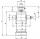Cylinder was drawn in scale 2:1. How many times is the volume of the cylinder smaller in reality?
• Cats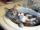Two cats caught two mice in two days. How many mouses will catch 6 cats for 6 days?
• Seamstress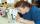Seamstress bought two kinds of textile together 25 meters. One at 50 SKK and the second 70 SKK per meter. How many meter bought from this two textiles when paid totally 1540 SKK?
• Shelf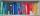How many ways are there to arrange 6 books on a shelf?
• Green - Red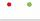We have 5 bags. Each consist one green and 2 red balls. From each we pull just one ball. What is the probability that we doesn't pull any green ball?
• The plan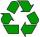Students have collected 48 kg of paper which is 16% of the plan. How many kg of paper still have to collect to fulfill the plan?
• Unit vector 2DDetermine coordinates of unit vector to vector AB if A[-6; 8], B[-18; 10].
• Trapezoid - hard exampleBase of the trapezoid are: 24, 16 cm. Diagonal 22, 26 cm. Calculate its area and perimeter.
• Homework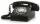The monthly fee for the phone is 12 USD and for phone unit 0.08 USD. How much family pay phone in the first quarter Q1, if on January 1 had on the counter 97362 units and on April 1 97946 units.
• Cone and the ratioRotational cone has a height 23 cm and the ratio of the base surface to lateral surface is 7: 9. Calculate a surface of the base and the lateral surface.
• Above EarthTo what height must a boy be raised above the earth in order to see one-fifth of its surface.
• Math classificationIn 3A class are 27 students. One-third got a B in math and the rest got A. How many students received a B in math?
• Axial sectionAxial section of the cylinder has a diagonal 31 cm long and we know that the area of the side and the area of base is in ratio 3:2. Calculate the height and radius of the cylinder base.
• Relay-race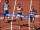Kate ran 170 meters at 29.8 seconds, Suzan at 29.3 seconds, Roza at 34 seconds and Žofka at 30.4 seconds. How long took to run 4x170 m relay-race?
• Hexagonal pyramidCalculate the volume and the surface of a regular hexagonal pyramid with a base edge length of 3 cm and a height of 5 cm.
• Rhombus and inscribed circleIt is given a rhombus with side a = 6 cm and the radius of the inscribed circle r = 2 cm. Calculate the length of its two diagonals.
• TimeageSeven times of my age is 8 less than the largest two-digit number. How old I am?

Do you have an interesting mathematical word problem that you can't solve it? Submit a math problem, and we can try to solve it.

We will send a solution to your e-mail address. Solved examples are also published here. Please enter the e-mail correctly and check whether you don't have a full mailbox.

Please do not submit problems from current active competitions such as Mathematical Olympiad, correspondence seminars etc...